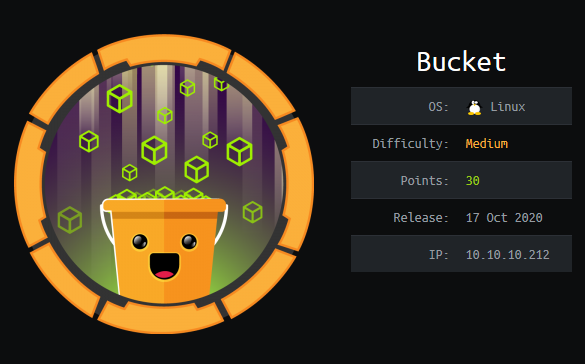Posts
Writeups
Cancel

# Script Kiddie

Given source file from Crypto.Cipher import AES import hashlib import secrets def header(): print(""" _____ _ __ __ _ | __ \(_)/ _|/ _(_) | | | |_| |_| |_ _ ___ | | | | | _| _| ...

This is an easy challenge. The prime is small so we can just brute force it. Just run through every possible int from 1 to p, square them and check if there are equal to the numbers. If they are th...

# Legendre Symbol

This makes you create a function to calculate if a number is a quadratic root faster. The math is pretty simple so here is the code for the function. NB: since pow() returns a positive interger and...

# Lazy CBC

Source code from Crypto.Cipher import AES KEY = ? FLAG = ? @chal.route('/lazy_cbc/encrypt/<plaintext>/') def encrypt(plaintext): plaintext = bytes.fromhex(plaintext) if len(plain...

# RSA Common Modulus

Common modulus attack In this case imagine that Alice sent the SAME message more than once using the same public key but thanks to the laws of the world, a problem happened and the public key chan...

# RSA Multiple Recipients

Hastad Attack Let’s go over the math of the attack: Assume Bob sends the same message to multiple people using RSA with different modulus but the same e for all of the messages. Assume that this ...

# Phonebook

We see that there is a form. At the bottom we can see the username we are supposed to use. It doesn’t take a genius to see that the password is most likely the flag. While testing we see that we c...

# Heist# Bucket# LostKey

Source code and output #!/usr/bin/env python3 from Crypto.Util.number import * from hashlib import sha1 from Crypto.Cipher import AES from Crypto.Util.Padding import pad from secret import flag, n...

Recent Update
Trending Tags